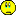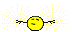# priority_queueدرSTL

#### naseri

##### New Member
سلام!

من می خوام از PRIORITY_QUEUEدر پیاده سازی الگوریتم کوله پشتی0/1 B&B استفاده کنم.(++borland c

ولی کار با STLرو بلد نیستم.<QUEUE.H>رو INCLUDE کردم.بعد نوشتمrority_queue<int> Q; اما کلا error میگیره.مشخصات template رو هم نمیدونم چی جوری باید بنویسم .

کسی میتونه یه تیکه ساده برام بنویسه؟ چند تا از اینترنت گرفتم ولی نمی فهمم.

#### saalek110

##### Well-Known Member
با سلام.
من بورلند سی را نصب کردم و از help آن مثال زیر را پیدا کردم که بی خطا اجرا شد:
کد:
``````#include <queue>

#include <deque>
#include <vector>

#include <string>
using namespace std;

int main(void)

{
// Make a priority queue of int  using a deque container

priority_queue<int, vector<int>, less<int> > pq;

// Push a couple of values

pq.push(1);

pq.push(2);

// Pop a couple of values and examine the ends

cout << pq.top() << endl;
pq.pop();
cout << pq.top() << endl;

pq.pop();

// Make a priority queue of strings

priority_queue<string,deque<string>, less<string> > pqs;

// Push on a few strings then pop them back off

int i;
for (i = 0; i < 10; i++)
{
pqs.push(string(i+1,'a'));
cout << pqs.top() << endl;
}
for (i = 0; i < 10; i++)
{
cout << pqs.top() << endl;
pqs.pop();

}

// Make a priority queue of strings using greater

priority_queue<string,deque<string>, greater<string> > pgqs;

// Push on a few strings then pop them back off

for (i = 0; i < 10; i++)
{
pgqs.push(string(i+1,'a'));
cout << pgqs.top() << endl;

}

for (i = 0; i < 10; i++)

{
cout << pgqs.top() << endl;
pgqs.pop();

}

return 0;

}``````

کتاب زیر هم شاید مفید باشد:
3 مگا.نقل از این کتاب:

10.3 Priority Queues
The class priority_queue<> implements a queue from which elements are read according to their priority. The interface is similar to queues. That is, push() inserts an element into the queue, whereas top() and pop() access and remove the next element (Figure 10.5). However, the next element is not the first inserted element. Rather, it is the element that has the highest priority. Thus, elements are partially sorted according to their value. As usual, you can provide the sorting criterion as a template parameter. By default, the elements are sorted by using operator < in descending order. Thus, the next element is always the "highest" element. If more than one "highest" element exists, which element comes next is undefined.Priority queues are defined in the same header file as ordinary queues, <queue>:

کد:
``#include <queue>``
In <queue>, the class priority_queue is defined as follows:
کد:
``````   namespace std {
template <class T,
class Container = vector<T>,
class Compare = less<typename Container::value_type> >
class priority_queue;
}``````
The first template parameter is the type of the elements. The optional second template parameter defines the container that is used internally by the priority queue for its elements. The default container is a vector. The optional third template parameter defines the sorting criterion that is used to find the next element with the highest priority. By default, it compares the elements by using operator <.

For example, the following declaration defines a priority queue of floats
کد:
``````   priority_queue<vector<float>,less<float> > buffer;

std::priority_queue<float> pbuffer;      // priority queue for floats``````
The priority queue implementation simply maps the operations into appropriate calls of the container that is used internally. You can use any sequence container class that provides random access iterators and the member functions front(), push_back(), and pop_back(). Random access is necessary for sorting the elements, which is performed by the heap algorithms of the STL (the heap algorithms are described in Section 9.9.4,). For example, you could also use a deque as the container for the elements:
کد:
`` std::priority_queue<float,std::deque<float> > pbuffer;``
To define your own sorting criterion you must pass a function or function object as a binary predicate that is used by the sorting algorithms to compare two elements (for more about sorting criteria, see Section 6.5.2, and Section 8.1.1,). For example, the following declaration defines a priority queue with reverse sorting:
کد:
``````   std::priority_queue<float,std::vector<float>,
std::greater<float> > pbuffer;``````
In this priority queue the next element is always one of the elements with the lowest value.

ولی مثال ادامه مطلب بالا یعنی کد زیر:
کد:
``````//10.3.2 Example of Using Priority Queues
//The following program demonstrates the use of class priority_queue<>:

// cont/pqueue1. cpp

#include <iostream>
#include <queue>
using namespace std;

int main()
{
priority_queue<float> q;

// insert three elements into the priority queue
q.push(66.6);
q.push(22.2);
q.push(44.4);

// read and print two elements
cout << q.top() << ' ';
q.pop();
cout << q.top() << endl;
q.pop();

// insert three more elements
q.push(11.1);
q.push(55.5);
q.push(33.3);

// skip one element
q.pop();

//pop and print remaining elements
while (!q.empty()) {
cout << q.top() << ' ';
q.pop();
}
cout << endl;
}``````
خطا داد.
ولی در یک پروژه کنسولی در ویژوال سی 6 با کد زیر:
کد:
``````// q.cpp : Defines the entry point for the console application.
//

#include "stdafx.h"
#include <iostream>
#include <queue>
using namespace std;

int main(int argc, char* argv[])
{

priority_queue<float> q;

// insert three elements into the priority queue
q.push(66.6);
q.push(22.2);
q.push(44.4);

// read and print two elements
cout << q.top() << ' ';
q.pop();
cout << q.top() << endl;
q.pop();

// insert three more elements
q.push(11.1);
q.push(55.5);
q.push(33.3);

// skip one element
q.pop();

//pop and print remaining elements
while (!q.empty()) {
cout << q.top() << ' ';
q.pop();
}
cout << endl;
return 0;
}``````
با 5 وارنینگ زیر:
کد:
``````F:\q\q.cpp(15) : warning C4305: 'argument' : truncation from 'const double' to 'const float'
F:\q\q.cpp(16) : warning C4305: 'argument' : truncation from 'const double' to 'const float'
F:\q\q.cpp(17) : warning C4305: 'argument' : truncation from 'const double' to 'const float'
F:\q\q.cpp(26) : warning C4305: 'argument' : truncation from 'const double' to 'const float'
F:\q\q.cpp(28) : warning C4305: 'argument' : truncation from 'const double' to 'const float'``````
اجرا شد.
پس احتمالا این کتاب برای وی سی 6 مناسب است.

•naseri

#### naseri

##### New Member
سلام!

ممنون از راهنمایی کامل شما.

چون میخوام الگوریتم رو گرافیکی پیاده کنم توی برلند همش errorمیگیره که BGIرو supportنمی کنه

هر بلایی هم سرش اوردم درست نشد!(بماند که helpاون هم کار نمیکنه!!)

اما توی turbo C++ این error رو نمیگیره ولی متاسفانه turbo من dequeue.h وvector.h وqueue.h رو includeنمیکنه!!

چیزی نشد.

سوال دوم اینکه من میخوام یه struct رو بزارم توی صف که 4 تا field داره که این صف باید بر اساس یکیشون sort بشه , و 3 تای دیگه پس از

pop کردن node با بالاترینpriority تغییرکنن .ایا امکان داره؟

آخرین ویرایش:

#### naseri

##### New Member
فکر نمی کنم امکان پذیر باشه.

باید از دو تا container استفاده کرد.حالا مساله حفظ ارتباط بین این دو است؟#### saalek110

##### Well-Known Member
فایلهای:
QUEUES.H
QUEUE.H
PRIORTYQ.H
در توربو سی 3 در پوشه:
C:\TC\CLASSLIB\INCLUDE
قرار دارد.
----------------------
پاسخ پست 4 :
احتمالا ممکنه برای این کار هم کلاسهایی در آن هدرها باشد. شاید هم نباشد.
برای حفظ ارتباط .....
آیا میشه؟ من روشش را نمی دانم.
سرچ کنید کلمات struct و queue را. به احتمال زیاد کسی قبلا این کار را کرده.

•naseri